# HC Verma Solutions Class 12 Chapter 6 Heat Transfer

HC Verma Solutions Class 12 Chapter 6 Heat Transfer is a valuable study tool that will enable students to learn comprehensively about all the concepts of heat transfer and related terms like radiation, conduction, and convection. The chapter also includes topics like thermal conduction, Prevost theory of exchange, blackbody radiation,  etc.  Students will find questions and exercises based on these topics.

HC Verma Solutions will thus suffice the need of students to know the right answers and understand the topic in depth. They will also develop an efficient problem-solving skill that is required to tackle complex physics questions given in chapter 6. All in all, students can use the solutions to take their preparation to a higher level especially for exams like JEE Main and Advanced.

## Key Topics Related To Heat Transfer

• Kirchhoff’s Law
• Newton’s Law of cooling
• Stefan Boltzmann Law
• Detection and Measurement of Radiation
• Series and Parallel Connection of Rods

## Class 12 Important Questions In Chapter 6

1. On a hot sunny day is it possible to cool a room by closing all the windows, doors and opening the refrigerator? Is the process practical?

2. The temperature in the atmosphere is around 600°C but any living being would instead freeze to death and not boil. Give valid reasons as to why this occurs?

3. Let’s take two objects O and P having equal surface areas and keep their temperatures at 10°C and 20°C respectively. Can you state what will the ratio of thermal radiation emitted in a given time by O and P be?

(a) 1 : 2 fa) 1 : 4 (c) 1 : 1.15 (d) 1 : 16

4. What special case according to you is Newton’s law of cooling?

(a) Stefan’s law (b) Planck’s law (c) Kirchoff’s law (d) Wien’s displacement law

5. What will happen to a blackbody when it comes in contact with radiation?

## HC Verma Solutions Vol 2 Heat Transfer Chapter 6

Question 1: A uniform slab of dimension 10 cm × 10 cm × 1 cm is kept between two heat reservoirs at temperatures 10°C and 90°C. The larger surface areas touch the reservoirs. The thermal conductivity of the material is 0.80 W m–1 °C–1. Find the amount of heat flowing through the slab per minute.

Solution:

Height of the slab : x = 1 cm = 0.01 m

Area of the uniform slab: A = 100 cm2 = 0.01 m2

ΔT = 90-10=80° C and K=0.80 W m–1 °C–1.

We know, Rate of amount of heat flowing,

Δθ/Δt = KAΔT/x = [0.80×0.01×80]/0.01 = 64 J/s = 3840 j/min

The amount of heat flowing through slab is 3840 J/min.

Question 2: A liquid-nitrogen container is made of a 1 cm thick Styrofoam sheet having thermal conductivity 0.025 J s–1m–1°C–1. Liquid nitrogen at 80 K is kept in it. A total area of 0.80 m2 is in contact with the liquid nitrogen. The atmospheric temperature is 300 K. Calculate the rate of heat flow from the atmosphere to the liquid nitrogen.

Solution:

Thickness of the container : x = 1 cm = 0.01 m

Thermal conductivity of the sheet : K= 0.025 J s–1m–1°C–1

Area of the container : A = 0.8 m2

Temperature of the liquid nitrogen: T1 = 80 K

Temperature of the atmosphere: T2 = 300 K

ΔT = T2 – T1 = 220 K

We know, Rate of amount of heat flowing,

Δθ/Δt = KAΔT/x = [0.025×0.8×220]/0.01 = 440 J/s

Question 3: The normal body-temperature of a person is 97°F. Calculate the rate at which heat is flowing out of his body through the clothes assuming the following values. Room temperature = 47°F, surface of the body under clothes = 1.6 m2, conductivity of the cloth = 0.04 J s–1 m–1 °C–1, thickness of the cloth = 0.5 cm.

Solution:

T1 = 97 °F = 36.1 °C ; T2 = 47°F = 8.3 °C

=> ΔT = T1 – T2 = 36.1 – 8.3 = 27.8 °C

Thickness of the cloth : x = 0.5 cm = 0.005 m

A = 1.6 m2 and K =0.04 J s–1 m–1 °C–1

We know, Rate of amount of heat flowing,

Δθ/Δt = KAΔT/x = [0.004×1.6×27.8]/0.005 = 355.84 J/s

Question 4: Water is boiled in a container having a bottom of surface area 25 cm2, thickness 1.0 mm and thermal conductivity 50W m–1 °C–1. 100g of water is converted into steam per minute in the steady state after the boiling starts. Assuming that no heat is lost to the atmosphere, calculate the temperature of the lower surface of the bottom.Latent heat of vaporization of water =2.26 × 106 J kg–1.

Solution:

Thickness of the container: x = 1 mm = 0.001 m

A = 25 cm2 = 0.0025 m2

K =50 W m–1 °C–1

L = 2.26 × 106 J kg–1

m = 100 g = 0.1 kg and T2 = 100 ° C

We know, Rate of amount of heat flowing,

Δθ/Δt = K AΔT/x

Also, Δθ = Q =L× m

=> (L× m)/Δt = K AΔT/x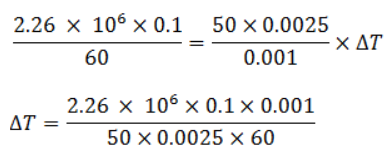T1 – T2 =30.13 + 100

=> T1 = 130.13 oC

Temperature of the bottom of the container is 130.13 ° C.

Question 5: One end of a steel rod (K = 46 J s–1 m–1 °C–1) of length 1.0 m is kept in ice at 0°C and the other end is kept in boiling water at 100°C. The area of cross-section of the rod is 0.04 cm2. Assuming no heat loss to the atmosphere, find the mass of the ice melting per second. Latent heat of fusion of ice = 3.36 × 105 J kg–1.

Solution:

Length of the rod = l = 1 m

K = 46 J s–1 m–1 °C–1

T1 = 100° C ; T2 = 0° C => ΔT = 100

A = 0.04 cm2 = 0.04× 10-4 m2

Latent heat of fusion of ice = 3.36 × 105 J kg–1

Rate of transfer heat:

Δθ/Δt = KAΔT/l = [46×0.04× 10-4x100]/1

= 184 x 104 J/s

Also, Δθ = m Lf

=> m x 3.36 x 105 = 184 x 10-4

=> m = 5.5 x 10-9 kg/s = 5.5 x 10-5 g/s

Question 6: An icebox almost completely filled with ice at 0°C is dipped into a large volume of water at 20°C. The box has walls of surface area 2400 cm2, thickness 2.0 mm and thermal conductivity 0.06 W m–1 °C–1. Calculate the rate at which the ice melts in the box. Latent heat of fusion of ice = 3.4 × 105 J kg–1.

Solution:

A = 2400 cm2 = 0.24 m2

T1 = 20° C and T2= 0° C

Thickness of the box = x = 2 mm = 0.002 m

K = 0.06 W m–1 °C–1

Latent heat of fusion of ice = L = 3.4 × 105 J kg–1

Rate of transfer heat:

Δθ/Δt = KAΔT/x = [46×0.04× 10-4x100]/1

Also, Δθ = Q = L× m

Substituting values, we get

L x Δm/Δt = 144

=> Δm/Δt = 4.23 x 10-4 kg/s

Using L = 3.4 × 105 J kg–1

Question 7: A pitcher with 1 mm thick porous walls contains 10 kg of water. Water comes to its outer surface and evaporates at the rate of 0.1 g s–1. The surface area of the pitcher (one side) = 200 cm2. The room temperature = 42°C, latent heat of vaporization = 2.27 × 106 J kg–1, and the thermal conductivity of the porous walls = 0.80 J s–1m–1 °C–1. Calculate the temperature of water in the pitcher when it attains a constant value.

Solution:

m = 10 kg

A = 200 cm2 = 0.02 m2.

Thickness of the pitcher: x = 1 mm = 0.001 m

Rate at which water evaporates at it’s outer surface:= 0.1 gs–1 = 0.1× 10-3 kg s–1.

T1 = 42 ° C

L = 2.27 × 106 J kg–1 and K = 0.80 J s–1m–1 °C–1.

Rate of transfer heat:

Δθ/Δt = KAΔT/x

Also, Δθ = Q = L× m

Now, 0.1× 10-3 kg of water evaporates in 1 second. Thus by unitary method, 10 kg of water will evaporate in 105 seconds.

Δt = 105 seconds.

=> (Lx m)/Δt = KAΔT/x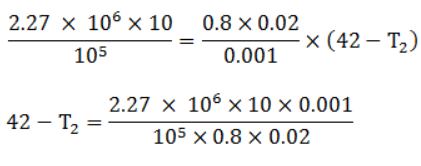42 – T2 = 14.18

=> T2 = 27.82 oC

Question 8: A steel frame (K = 45 W m–1 °C–1) of total length 60 cm and cross-sectional area 0.20 cm2, forms three sides of a square. The free ends are maintained at 20°C and 40°C. Find the rate of heat flow through a cross-section of the frame.

Solution:

Length of the steel frame : x = 60 cm = 0.6 m

K=45 W m–1 °C–1.

Area of cross section : A = 0.20 cm2 = 0.2 × 10-4 m2.

ΔT = 40 – 20= 20°C.

Rate of transfer heat:

Δθ/Δt = KAΔT/x = [45×0.2×10-4x20]/[0.6] = 0.03 J/s

Question 9 : Water at 50°C is filled in a closed cylindrical vessel of height 10 cm and cross-sectional area 10 cm2. The walls of the vessel are adiabatic but the flat parts are made of 1 mm thick aluminium (K = 200 J s–1 m–1 °C–1). Assume that the outside temperature is 20°C. The density of water is 1000 kg m–3, and the specific heat capacity of water = 4200 J k–1 m–1 °C–1. Estimate the time taken for the temperature to fall by 1.0°C. Make any simplifying assumptions you need but specify them.

Solution: Height of the vessel : h = 10 cm = 0.1 m

Cross section area of the vessel: A = 10 cm2 = 0.001 m2

x = 1 mm = 0.001 m and K =200 J s–1 m–1 °C–1

T1 = 50 °C and T2 = 20 °C => ΔT = 30°C

Density(ρ) = mass/volume = 1000 kg m-3 . ….(1)

specific heat capacity of water = 4200 J k–1 m–1 °C–1

Rate of amount of heat flowing:

Δθ/Δt = KAΔT/x = [2x 200×0.001×30]/[0.001] = 12000 J/s …(2)

Also, we know that, ΔQ = Δθ = msΔT

=> Δθ/Δt = msΔT/Δt …(3)

From (1), mass = 1000 x 0.001 x 0.1 = 0.1 kg …(4)

Now, find time taken for the temperature to drop by 1°C .

using (3) and (4) in (2), we get

12000 = [0.1x4200x1]/Δt

=> Δt = t = 0.035 s

Therefore, it took around 0.035 seconds for the temperature to drop by 1°C.

Question 10: The left end of a copper rod (length = 20 cm, area of cross-section = 0.20 cm2) is maintained at 20°C and the right end is maintained at 80°C. Neglecting any loss of heat through radiation, find

(a) the temperature at a point 11 cm from the left end and

(b) the heat current through the rod. Thermal conductivity of copper = 385 W m–1 °C–1.

Solution:

Length of the rod: x = 20 cm =0.2 m

Area of cross section of the rod = A = 0.2 cm2 = 0.2× 10-4 m2

T1 = 80° C and T2 = 20° C

Thermal conductivity of copper: K = 385 W m–1 °C–1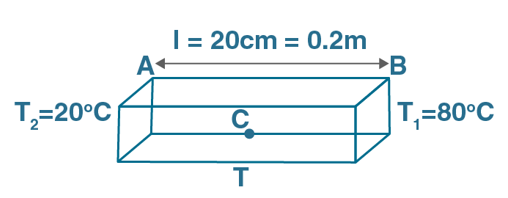Let’s first solve for part (b)

Rate of flow of heat = [temp differences]/thermal resistance]

ΔQ/Δt = KAΔT/x

On substituting the values, we get

ΔQ/Δt = 2310 x 10-3 = 2.31 J/s

(a) Let “T” be the temperature at a point 11 cm from the left end .

Now, ΔQ/Δt = KAΔT/x = KA(T-20)/x

=> T – 20 = [2.31x 11 x 10-2]/[383×0.2×10-4] = 33

=> T = 33 + 20 = 53oC

Question 11: The ends of a metre stick are maintained at 100°C and 0°C. One end of a rod is maintained at 25°C. Where should its other end be touched on the metre stick so that there is no heat current in the rod in steady state?

Solution:

Here, ΔT = T2 – T1 = 100-0 = 100 °C

Length of the rod = l = 1 m

Let Temperature of one end of the rod = T3 = 25 °C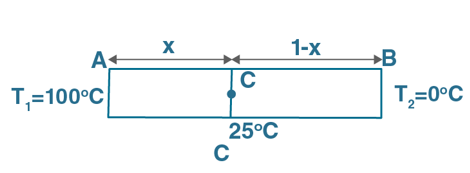Distance between A and C = x

Distance between C and B = 1 – x

Rate of amount of heat flowing:

Δθ/Δt = KAΔT/x

For zero heat current in the rod, the temperature difference must be zero i.e.ΔT = 0.

Heat current between A and C must be equal to the heat current between C and B since one end of the rod is maintained at 25 °C, the other end must be maintained at 25 °C.

(Δθ/Δt)AC = (Δθ/Δt)CB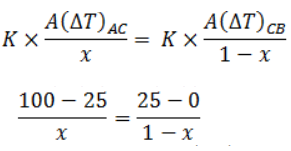=> 75 – 75x = 25x

=> x = 0.75 m

Question 12: A cubical box of volume 216 cm3 is made up to 0.1 cm thick wood. The inside is heated electrically by a 100 W heater. It is found that the temperature difference between the inside and the outside surface is 5°C in steady state. Assuming that the entire electrical energy spent appears as heat, find the thermal conductivity of the material of the box.

Solution:

Volume of the box : V = 216 cm3 = 216× 10-6 m3

Let “a” be the side of a cube, then a3 = 216× 10-6

=> a = 0.06 m

So, Surface area of the cube = A= 6a2

=> A = 6× (0.06)2 = 0.0216 m2.

Temperature difference = ΔT = 5 °C

x = 0.1 cm = 0.001 m

Power of the heater = P = 100 W

Rate of amount of heat flowing:

Δθ/Δt = KAΔT/x …(1)

We know that,Power = Energy per unit time

=> Δθ/Δt = P = 100 W

(1)=> 100 = K x [0.0216 x 5]/0.001

=> K = 0.9259 W/moC

Thus, thermal conductivity of the box is 0.9259 W/moC

Question 13: Figure shows water in a container having 2.0 mm thick walls made of a material of thermal conductivity 0.50 W m–1 °C–1. The container is kept in a melting ice bath at 0°C. The total surface area in contact with water is 0.05 m2. A wheel is clamped inside the water and is coupled to a block of mass M as shown in the figure. As the block goes down, the wheel rotates. It is found that after some time a steady state is reached in which the block goes down with a constant speed of 10 cm s–1 and the temperature of the water remains constant at 1.0°C. Find the mass M of the block. Assume that the heat flows out of the water only through the walls in contact. Take g = 10 m s–2.Solution:

Thickness of the container: x = 2 mm = 0.002 m

Thermal conductivity of the container: K= 0.50 W m–1 °C–1

T1 = 1 °C and T2 = 0 °C

Speed of the block = v = 10 cm/s = 0.1 m/s

Now,

Rate of amount of heat flowing: Δθ/Δt = KAΔT/x

The effect of the block going down and the heat transfer has one identity in common, which is power.

We know, P = W/t

Therefore, P = Fd/t = Fv

Here, W is the work done by the block = P = mgv

=> P = mx 10 x 0.1 = m

Also, in terms of Heat Energy: P= Energy per unit time

=> P = Δθ/Δt = KAΔT/x

or M = KAΔT/x = [0.50×0.05×1]/0.002 = 12.5 Kg

Which is the mass of the block.

Question 14: On a winter day when the atmospheric temperature drops to –10°C, ice forms on the surface of a lake.

(a) Calculate the rate of increase of thickness of the ice when 10 cm of ice is already formed.

(b) Calculate the total time taken in forming 10 cm of ice. Assume that the temperature of the entire water reaches 0°C before the ice starts forming. Density of water = 1000 kg m–3, latent heat of fusion of ice = 3.36 × 105 J kg–1 and thermal conductivity of ice = 1.7 W m–1 °C–1. Neglect the expansion of water on freezing.

Solution:

Length of the ice formed = l = 10 cm = 0.1 m

Latent heat of fusion of ice = L = 3.36 × 105 J kg–1

Thermal conductivity of ice = K = 1.7 W m–1 °C–1

Density of water = ρ = 1000 kg m–3

T1 = 0 °C and T2 = -10 °C

So, ΔT = T1 – T2 = 10 °C

Now,

Rate of amount of heat flowing: Δθ/Δt = KAΔT/x …(1)

Also, Δθ = Q = Lm …(2)

Where, Q = amount of heat absorbed or released

L = Latent heat and

m = mass of the substance

Also, we know, density(ρ) = mass(m) x volume(v)

or m = ρ V = ρAl …(3)

(a)Let change in thickness be Δx, thus rate of change of thickness:

Δx/Δt = KAΔT/Δθ from (1)

=> Δx/Δt = KAΔT/(ρAl)L

[Using (2) and (3)]

=> Δx/Δt = [1.7×10]/[0.1×3.36x1000x105] = 5.05×10-7 m/s

Thickness of the ice increases at a rate of 5.05× 10-7 m/s.

(b) Consider time required to form thin layer of ice dx is dt.

And heat absorbed by the thin layer, dQ = dmL

Now, the rate of Heat transfers due to thin layer:

(1)=> L dm/dt = KAΔT/x

=> LAρ dx/dt = KAΔT/x

=> dt = [Lρx dx]/[kΔT]

Integrating above equation from range x = 0 to 0.1, we get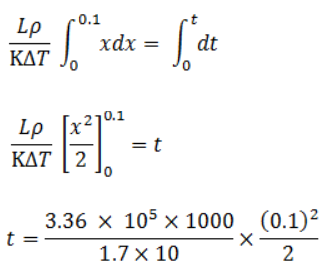=> t = 98823.52/3600 = 27.45 hours

Hence, it took 27.45 hours to form 10 cm thick ice.

Question 15: Consider the situation of the previous problem. Assume that the temperature of the water at the bottom of the lake remains constant at 4°C as the ice forms on the surface (the heat required to maintain the temperature of the bottom layer may come from the bed of the lake). The depth of the lake is 1.0 m. Show that the thickness of the ice formed attains a steady state maximum value. Find this value. The thermal conductivity of water = 0.50 Wm-1 °C-1. Take other relevant data from the previous problem.

Solution:

Depth of the lake: d = 1 m

Thermal conductivity of water: KW = 0.50 Wm-1 °C-1

Thermal conductivity of ice: K_I = 1.7 W m-1 °C-1

T1 = 4 °C and T2 = – 10 °C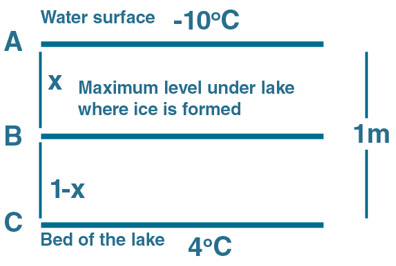Distance AB is : xAB = x m

Distance CB is : xCB = (1 – x) m

Now,

Rate of amount of heat flowing: Δθ/Δt = KAΔT/x

In the diagram, point B depicts the maximum level upto which ice can be formed inside the lake.

Temperature at B : T3 = 0°C

Ice attains a steady state maximum level, so temperature difference between points A,B and C would be unchanged.

The rate of heat transfer between A and B equals the rate of heat transfer between B and C.

Here, x is the thickness or length of the material. Find x.

(Δθ/Δt)_CB = (Δθ/Δt)_AB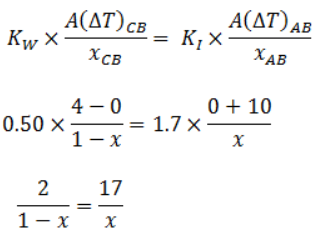=> x = 0.894 m

Question 16: Three rods of lengths 20 cm each and area of cross-section 1 cm2 are joined to form a triangle ABC. The conductivities of the rods are KAB = 50 J s-1 m-1 °C-1, KBC = 200 J s-1 m-1 °C-1 and KAC = 400 J s-1 m-1 °C-1. The junctions A, B and C are maintained at 40°C, 80°C and 80°C respectively. Find the rate of heat flowing through the rods AB, AC and BC.

Solution:

Length of all the rods: x= AB=BC=AC = 20 cm = 0.2 m

Area of cross section of these rods: A = 1 cm2 = 0.0001 m2

Thermal conductivity of rod AB : KAB = 50 J J s-1 m-1 °C-1

Thermal conductivity of rod BC : KBC = 200 J s-1 m-1 °C-1

Thermal conductivity of rod AC : KAC = 400 J s-1 m-1 °C-1

Temperature at A : T1 = 40 °C

Temperature at B : T2 = 80 °C

Temperature at C : T3 = 80 °C

Now,

Rate of amount of heat flowing: Δθ/Δt = KAΔT/x

Rate of heat flowing in the rod AB: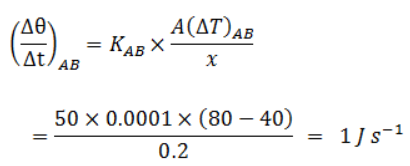Rate of heat flowing in the rod BC: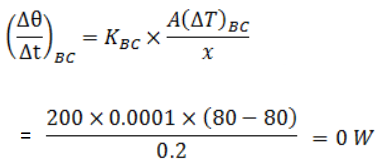Rate of heat flowing in the rod BC: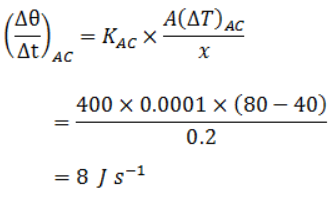Question 17: A semicircular rod is joined at its end to a straight rod of the same material and the same cross-sectional area. The straight rod forms a diameter of the other rod. The junctions are maintained at different temperatures. Fid the ratio of the heat transferred through a cross-section of the semicircular rod to the heat transferred through a cross-section of the straight rod in a given time.

Solution:

Temperature at junction 1: T1

Temperature at junction 2: T2

Length of the rod 1 = x= d=2r

Length of the rod 2 = x’ and circumference = πr

Now,

Rate of amount of heat flowing: Δθ/Δt = KAΔT/x

As both the rods have same material, their thermal conductivity is the same.

Rate of heat flowing through rod 1 and rod 2 be: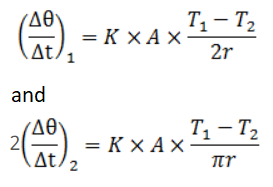Ratio of the rate of heat transferred from semi circular rod to straight rod is: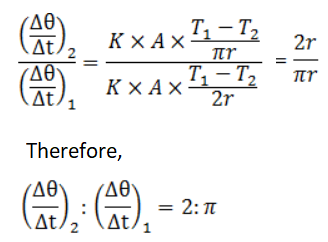Question 18: A metal rod of cross-sectional area 1.0 cm3 is being heated at one end. At one time, the temperature gradient is 5.0°C cm–1 at cross-section A and is 2.5°C cm–3 at cross-section B. calculate the rate at which the temperature is increasing in the part AB of the rod. The heat capacity of the part AB = 0.40 J°C–1, thermal conductivity of the material of the rod = 200 W m–1 °C–1. Neglect any loss of heat to the atmosphere.

Solution:

Cross sectional area of the metal rod: A = 1 cm2 = 0.0001 m2

Temperature gradient at A = (dT/dx)A = 5.0 °C cm–1 = 500 °C m–1

Temperature gradient at B = (dT/dx)B = 250 °C m–1

Heat capacity of the rod AB = C = 0.40 J°C–1

Thermal conductivity of the material of the rod, K = 200 W m–1 °C–1

Now,

Rate of amount of heat flowing: Δθ/Δt = KAΔT/x

Rate of heat flow is dθ/dt. So, at cross section A:

(dθ/dt)A = KA (dT/dx)A = 200 x 0.0001×500 = 10 J/s

At cross section B:

(dθ/dt)B = KA (dT/dx)B = 200 x 0.0001×250 = 5 J/s

Now,

Rate of flow of heat throughout the rod AB:

Δθ/Δt = (dθ/dt)A – (dθ/dt)B

= 10 -5 = 5 J/s

Also, we know Δθ = Q = msΔT And Heat Capacity= C = ms = Q/ΔT

=> Δθ/Δt = msΔT/Δt

=> 5 = 0.4 x ΔT/Δt

=> ΔT/Δt = 12.5 oC/s

Which is the required rate at which temperature increases.

Question 19: Steam at 120°C is continuously passed through a 50 cm long rubber tube of inner and outer radii 1.0 cm and 1.2 cm. The room temperature is 30°C. Calculate the rate of heat flow through the walls of the tube. Thermal conductivity of rubber = 0.15 J s–1 m–1 °C–1.

Solution:

Steam temperature = T1 = 120 °C

Length of the tube = l = 50 cm = 0.5 m

Room temperature: T2 = 30 °C

Inner radii of the tube= r = 1 cm = 0.01 m

Outer radii of the tube = R = 1.2 cm = 0.012 m

Thermal conductivity of rubber: K = 0.15 J s–1 m–1 °C–1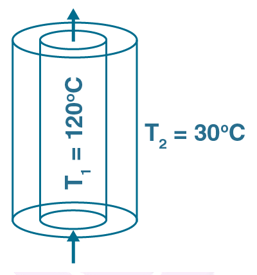Consider an element dx at a distance of x from the center between r and R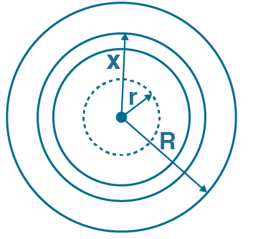Let us consider a strip at a distance r form the center of thickness dr.

dθ/dt = -(K 2π xldT/dx)

Here, x is the radius of the tube due to dx and l is the length of the tube.

or q x dx/x = -(2πlK)dT

[Heat flow can be given as q = Δθ/Δt]

Now we integrate both the sides, taking temperature from tube to surrounding: T1 to T2 and radii from r to R, we get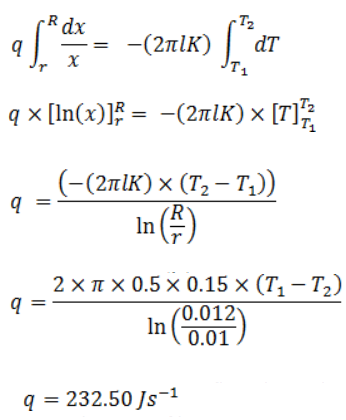Rate of heat flow through the walls of the tube is 232.50 J/s.

Question 20: A hole of radius r1 is made centrally in a uniform circular disc of thickness d and radius r2. The inner surface (a cylinder of length d and radius r1) is maintained at ta temperature θ1 and the outer surface (a cylinder of length d and radius r2) is maintained at a temperature θ21 > θ2). The thermal conductivity of the material of the disc is K. Calculate the heat flowing per unit time through the disc.

Solution:

Let r1 be the radius of the inner cylinder

Length of the cylinder = thickness of the disc = d

Temperature of inner cylinder and outer surface be = θ1 and θ2 respectively

Consider an imaginary cylinder of radius r and thickness dr between r1 and r2.

Here K be the thermal conductivity of the material of the disc.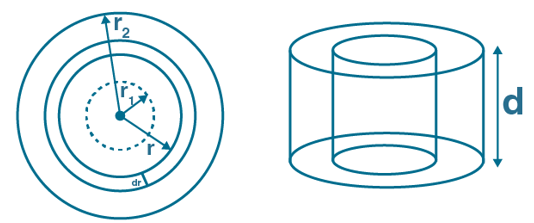Integrate the imaginary cylinder to get total heat transferred.

We know, differential form heat flow: dθ/dt = -(KAdT/dr) = q

Where, “q” is the rate of heat flowing.

-ve sign indicates the decrease in rate of heat flow with increase in the thickness of the imaginary tube.

A = area of the cylinder = 2πrd

=> q = -2πrdKdT/dr

=> q x dr/r = -2πrdK dT

Integrating both the sides by taking radius from r1 to r2 and temperature from θ1 to θ2.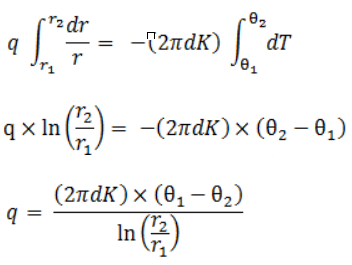Which is the heat flowing per unit time through the disc.